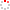NSSC OpenIR  > 空间科学部
 Bounce-averaged diffusion coefficients for superluminous wave modes in the magnetosphere Xiao, Fuliang; Chen, Liangxu; He, Yihua; Xiao, FL (reprint author), Changsha Univ Sci & Technol, Sch Phys & Elect Sci, Changsha 410004, Hunan, Peoples R China. Department 空间科学部 Source Publication JOURNAL OF ATMOSPHERIC AND SOLAR-TERRESTRIAL PHYSICS2011 Volume 73Issue:1Pages:88-94 ISSN 1364-6826 Language 英语 Keyword Wave-particle Interaction Superluminous Waves Bounce-averaged Diffusion Coefficients Abstract In this study, we present a first evaluation of bounce-averaged diffusion coefficients of superluminous wave (R-X and L-O) modes at two locations: L = 6.5 and 4.5. We show that, analogous to the local diffusion coefficients, bounce-averaged momentum diffusion coefficients play a dominant role for pitch angles alpha above a critical angle alpha(c), namely, < D(pp)> > vertical bar < D(p alpha)> vertical bar > < D(alpha alpha)>. We also demonstrate that for a Gaussian distribution of wave normal angle theta (X = tan theta). diffusion coefficients are sensitively dependent on the peak X(m). As X(m) increases, < D(pp)> and < D(alpha alpha)> are found to increase in cases of interest: X(m) = tan 25 degrees, tan 45 degrees and tan 65 degrees. We have estimated wave amplitudes for particular stochastic acceleration timescale for 1 MeV electrons and found that the required wave amplitudes B(te) similar to 86 to similar to 170 pT for a timescale tau = 1 day. These results indicate that superluminous wave modes may have a significant potential for both stochastic acceleration of trapped electrons (with higher pitch angles) and loss process of untrapped electrons (with smaller pitch angles). (C) 2009 Elsevier Ltd. All rights reserved.; In this study, we present a first evaluation of bounce-averaged diffusion coefficients of superluminous wave (R-X and L-O) modes at two locations: L = 6.5 and 4.5. We show that, analogous to the local diffusion coefficients, bounce-averaged momentum diffusion coefficients play a dominant role for pitch angles alpha above a critical angle alpha(c), namely, < D(pp)> > vertical bar < D(p alpha)> vertical bar > < D(alpha alpha)>. We also demonstrate that for a Gaussian distribution of wave normal angle theta (X = tan theta). diffusion coefficients are sensitively dependent on the peak X(m). As X(m) increases, < D(pp)> and < D(alpha alpha)> are found to increase in cases of interest: X(m) = tan 25 degrees, tan 45 degrees and tan 65 degrees. We have estimated wave amplitudes for particular stochastic acceleration timescale for 1 MeV electrons and found that the required wave amplitudes B(te) similar to 86 to similar to 170 pT for a timescale tau = 1 day. These results indicate that superluminous wave modes may have a significant potential for both stochastic acceleration of trapped electrons (with higher pitch angles) and loss process of untrapped electrons (with smaller pitch angles). (C) 2009 Elsevier Ltd. All rights reserved. Indexed By SCI ; EI Funding Project 中国科学院空间科学与应用研究中心 Document Type 期刊论文 Identifier http://ir.nssc.ac.cn/handle/122/3119 Collection 空间科学部 Corresponding Author Xiao, FL (reprint author), Changsha Univ Sci & Technol, Sch Phys & Elect Sci, Changsha 410004, Hunan, Peoples R China. Recommended CitationGB/T 7714 Xiao, Fuliang,Chen, Liangxu,He, Yihua,et al. Bounce-averaged diffusion coefficients for superluminous wave modes in the magnetosphere[J]. JOURNAL OF ATMOSPHERIC AND SOLAR-TERRESTRIAL PHYSICS,2011,73(1):88-94. APA Xiao, Fuliang,Chen, Liangxu,He, Yihua,&Xiao, FL .(2011).Bounce-averaged diffusion coefficients for superluminous wave modes in the magnetosphere.JOURNAL OF ATMOSPHERIC AND SOLAR-TERRESTRIAL PHYSICS,73(1),88-94. MLA Xiao, Fuliang,et al."Bounce-averaged diffusion coefficients for superluminous wave modes in the magnetosphere".JOURNAL OF ATMOSPHERIC AND SOLAR-TERRESTRIAL PHYSICS 73.1(2011):88-94.
 Files in This Item: File Name/Size DocType Version Access License 201173188.pdf（353KB） 开放获取 CC BY-NC-SA Application Full Text
 Related Services Recommend this item Bookmark Usage statistics Export to Endnote Google Scholar Similar articles in Google Scholar [Xiao, Fuliang]'s Articles [Chen, Liangxu]'s Articles [He, Yihua]'s Articles Baidu academic Similar articles in Baidu academic [Xiao, Fuliang]'s Articles [Chen, Liangxu]'s Articles [He, Yihua]'s Articles Bing Scholar Similar articles in Bing Scholar [Xiao, Fuliang]'s Articles [Chen, Liangxu]'s Articles [He, Yihua]'s Articles Terms of Use No data! Social Bookmark/Share